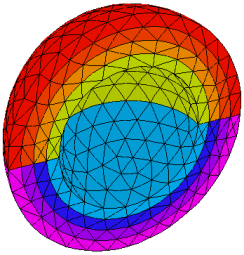﻿ Sample Problems > Usage > 3D_domains > 3d_shells

# 3d_shells

Navigation:  Sample Problems > Usage > 3D_domains >

# 3d_shells{ 3D_SHELLS.PDE    This problem demonstrates the construction  of multiple nested spherical shells.  We solve a heatflow equation with fixed  temperatures on inner and outer  shell surfaces. }   title 'Nested 3D Shells'   coordinates    cartesian3   variables    u   definitions    k = 10                heat =6*k            rad=sqrt(x^2+y^2)    R1 = 1    thick = 0.1      R2 = R1-thick    R3 = R2-thick    R4 = R3-thick    R5 = R4-thick   equations    U: div(K*grad(u)) + heat   = 0extrusion

surface 'SB1' z =  -SPHERE ((0,0,0),R1)     { the bottom hemisphere }

layer 'LB1'

surface 'SB2' z =  -SPHERE ((0,0,0),R2)

layer 'LB2'

surface 'SB3' z =  -SPHERE ((0,0,0),R3)

layer 'LB3'

surface 'SB4' z =  -SPHERE ((0,0,0),R4)

layer 'LB4'

surface 'SB5' z =  -SPHERE ((0,0,0),R5)

layer 'LB5'

surface 'ST5' z = SPHERE ((0,0,0),R5)

layer 'LT4'

surface 'ST4' z = SPHERE ((0,0,0),R4)

layer 'LT3'

surface 'ST3' z = SPHERE ((0,0,0),R3)

layer 'LT2'

surface 'ST2' z = SPHERE ((0,0,0),R2)

layer 'LT1'

surface 'ST1' z = SPHERE ((0,0,0),R1)       { the top hemisphere }

boundaries

surface 'SB1' value(u) = 0     { fixed values on outer sphere surfaces }

surface 'ST1' value(u) = 0

Region 1

layer 'LB1' k=1

layer 'LT1' k=1

start(R1,0)

value(u) = 0

arc(center=0,0) angle=180

natural(u)=0 line to close

Limited Region 2

layer 'LB2' k=2

layer 'LT2'  k=2

! include the region in all layers that must merge out:

layer 'LB3' layer 'LB4' layer 'LB5' layer 'LT4' layer 'LT3'

start(R2,0)

arc(center=0,0) angle=180

nobc(u) line to close

Limited Region 3

layer 'LB3'  k=3

layer 'LT3'  k=3

! include the region in all layers that must merge out:

layer 'LB4' layer 'LB5' layer 'LT4'

start(R3,0)

arc(center=0,0) angle=180

nobc(u) line to close

Limited Region 4

layer 'LB4'  k=4

layer 'LT4'  k=4

! include the region in all layers that must merge out:

layer 'LB5'

start(R4,0)

arc(center=0,0) angle=180

nobc(u) line to close

Limited Region 5

surface 'SB5' value(u) = 1   { fixed values on inner sphere surfaces }

surface 'ST5' value(u) = 1

layer 'LB5' void           { empty center }

start(R5,0)

arc(center=0,0) angle=180

nobc(u) line to close

monitors

grid(x,y,z)

grid(x,z) on y=0

contour(u) on x=0         { YZ plane through diameter }

contour(u) on y=0         { XZ plane through diameter }

contour(u) on z=0         { XY plane through diameter }

contour(u) on x=0.5       { YZ plane off center }

contour(u) on y=0.5       { XZ plane off center }

definitions

yp = 0.5

rp = sqrt(R2^2-yp^2)

xp = rp/sqrt(2+thick)

plots

grid(x,y,z)

grid(x,z) on y=0

contour(u) on x=0         as "Temp on YZ plane through diameter"

contour(u) on y=0         as "Temp on XZ plane through diameter"

contour(u) on z=0         as "Temp on XY plane through diameter"

contour(u) on z=0.001     as "Temp on XY plane through diameter"

contour(u) on x=0.5       as "Temp on YZ plane off center"

contour(u) on y=0.5       as "Temp on XZ plane off center"

contour(magnitude(grad(u))) on y=0.5 as "Flux on XZ plane off center"